# Dynamic Memory Allocation to Multidimensional Array Pointers

We already know why we need to allocate memory and how to allocate memory to a pointer variable. We have been discussing about dynamically allocating memory to a pointer variables, structures, and single dimensional arrays. Like any other pointers, when a pointer to a pointer is defined, we need to allocate memory to them too. There are several ways to allocate memory to double pointers. Let us discuss each of them below.

1     Using typedef keyword : This keyword is used to define a datatype for a variable. Suppose we want to declare a variable as array. Usually we declare it with normal notation as below:

`` int intArr ;``

This is a basic declaration which tells the compiler that it is an array of 10 integer values. Suppose we define few more arrays of same type. We can declare them in the same way as above. But there is a chance that latter we change the datatype of it to float or double or any other type. Then we need to scan the whole code to check if we have defined any other variable as array of same type and need to change it. But if we define a common user defined datatype for this array at once and then use them everywhere in the code to declare these array, then our task will be easier. That means, we define a common datatype as below for the array.

``    typedef int Arrays ;``

Above syntax defines user defined datatype Arrays which is an integer array of 10 elements. Now if we want to declare multiple arrays of 10 integer elements, then we can declare them as below:

`````` Arrays arrX; // declares an array of integer
Arrays arrY;``````

Now when we say a 2D array, intArr [] [] which is equivalent to writing *(intArr) []. Here *intArr points to the beginning of each row of the array, i.e.; intArr , intArr  etc. Hence if we use typedef to define these arrays of row elements it would as below:

`````` typedef int rowArrays ; // here 10 is the number of columns of the array
rowArrays *rowPtr; // this is same as declaring a single dimensional array of 10 elements``````

Hence rowPtr is array of array i.e.; *rowPtr is an array and it is declared as another array. Hence is an array of array which is 2D array. Thus we can allocate memory to rowPtr using malloc as shown below: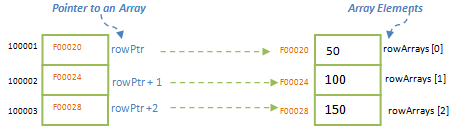``````#include <stdio.h>
#include <stdlib.h>

#define ROWS 3
#define COLS 5

int main ()
{
typedef int rowArrays [COLS]; // here 10 is the number of columns of the array
rowArrays *rowPtr; // this is same as declaring a single dimensional array of 10 elements

rowPtr = malloc (ROWS * COLS * sizeof (int));
rowPtr   = 10; // Now we can assign values to any element of the array
print f("Value of 4th column in the ma matrix is : %d\n", rowPtr);
return 0;
}
``````

The output of above program is as shown below: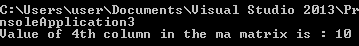Suppose we have not allocated memory to the pointers in above program (comment the malloc in above program and run the code). We will get below error message saying pointer variable is not initialized.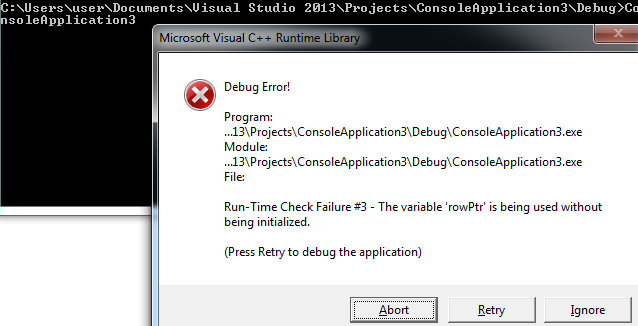One of the advantages of this method is that, though we have used pointer to declare, array notation can be used throughout the program to refer the elements pointed by it.  We can see that we have used rowPtr   instead of **rowPtr. It also helps in passing the array as arguments and gets modified, provided we need to pass number of columns (COLS) along with the array when we pass it as argument.

2.    In the above method, we used typedef to define the array and then we used it to define the pointer. Actually here typedef is not really necessary. Actual use of typedef can be found in some other section; above method simply defines one method of declaring a pointer. Hence we can write directly int *rowArr [COLS], instead of declaring it as rowArrays *rowArr; Rest of the memory allocation method remains the same as above.

3.    Most of the time, while using the pointers for arrays we will not know the actual size of the array. It will be decided at the run time. In such cases, memory is allocated to the pointers at the run time itself, depending upon the number of rows and columns. Below example gets the number of rows and columns as input and assigns the memory to the pointer.

``````#include <stdio.h>
#include <stdlib.h>

int main()
{
int intRow, intCol, index;
int **arrPtr; // 2D Array Pointer

printf("Enter the number of rows and columns for the array :");
scanf("%d", &intRow);
scanf("%d", &intCol);

arrPtr = malloc(intRow * sizeof(int *)); // Allocates memory for each row pointer
if (arrPtr == NULL) {
printf("Could not allocate memory to the row pointer");
exit(0);
}
for (index = 0; index< intRow; index++){
arrPtr[index] = malloc(intCol *sizeof(int)); // Allocate memory to each row to hold intCol columns
if (arrPtr[index] == NULL) {
printf("Could not allocate memory to the row %d", index);
exit(0);
}
}

arrPtr = 35; // Now we can assign values to any element of the array
printf("Value of 2nd column in the ma matrix is: %d\n", arrPtr);

return 0;
}
``````

In this example, we initially assign the memory to the pointer to pointer, arrPtr to hold intRow of row pointers, i.e.; we make arrPtr to hold the pointers to each row of the array which is again a pointer. Then we allocate memory to each row pointer, arrPtr [index] to hold columns in each row. Here we can notice that we need initial malloc call for assigning memory to row pointer; and then intRow subsequent malloc call for each row to get memory for its columns. Hence we need to call malloc for total of 1+intRow number of times to get memory allocated. Since we have allocated memory to row pointers first and then to its columns, we need not have all the columns of the array in contiguous memory locations. However we can use array notation here to refer any elements in the array as if they are in contiguous memory locations.

P.S: - In the first and second methods above, we have allocated memory in one call to malloc and their elements are in contiguous memory locations.

4.    In this method, we create an array and allocate memory to the whole array. Then we create another double pointer to point to each row in the array. i.e.; *arrPtr is created first (which is also considered as an array) and enough memory is allocated to it to hold both row and column elements. Then double pointer **rowPtr is created to point each row in *arrPtr, and memory is allocated to hold each row. We can see this in below example:

``````#include <stdio.h>
#include <stdlib.h>

int main ()
{
int *arrPtr; // Pointer to the whole array
int **rowPtr; // Row Pointer
int intRow = 5;
int intCol = 4;
int index=0;

arrPtr = malloc (intRow * intCol * sizeof (int)); // Allocates memory for whole array of intRow X intCol
if (arrPtr == NULL) {
printf ("Could not allocate memory to array");
exit (0);
}
rowPtr = malloc (intRow*sizeof (int *)); // Allocate memory for row pointers
if (rowPtr [index] == NULL) {
printf ("Could not allocate memory to row pointer");
exit (0);
}

// now make each row pointer to point to the columns
for (index = 0; index <intRow; index++) {
rowPtr [index] = arrPtr + (index * intCol);
}

rowPtr  = 35; // Now we can assign values to any element of the array
printf ("Value of 2nd column in the ma matrix is: %d\n", rowPtr  );

/*// Shows memory address at each row
printf ("\n index		rowPtr [index]		rowPtr [index] - rowPtr [index - 1]");
for (index = 0; index < intRow; index++) {// for each row
printf ("\n %d		%p", index, rowPtr [index]); // prints row number and starting address of that row
if (index > 0)
printf ("	%d", (rowPtr [index] – rowPtr [index - 1])); // number memory blocks allocated to each row
}*/
return 0;
}
``````

This method may look confusing at first. But let us try to understand what it does actually in the memory. We do not know the size of the array at compile time. intRow and intCol values are replaced at run time. Hence we need to allocate memory at run time itself. Here arrPtr is pointers which can hold an array and we need it hold as many records as two dimensional array holds. Hence allocate the memory to hold intRow X intCol i.e.; malloc (intRow * intCol * sizeof (int)). Now arrPtr is capable of storing elements for 2D array of size intRow X intCol. Memory allocated to it is in contiguous locations.
If we need to represent 2D array using array notation, we cannot use arrPtr which is a single pointer and can be used as single dimensional array. In order to represent a 2D array, we need a pointer to a pointer. Hence we declare **rowPtr. This also needs memory location, and we assign first memory to its row pointers, i.e.; malloc (intRow*sizeof (int *)). Now it will have some other memory location allocated to it. Our goal here is to have 2D array elements in contiguous memory locations while assigning memory at run time. Hence we will change the rowPtr memory to point to the memory locations that arrPtr is having (which is a contiguous memory address, i.e.; rowPtr [index] = arrPtr + (index * intCol). This code makes each row pointer to point to the contiguous memory address and allocates intCol of space to each row. Thus 2D array gets contiguous space at run time and could be accessed using array notation.

If one needs to be more clearly on the memory address uncomment the code above and execute the program to see the memory address at each row, and its total memory allocated to each row.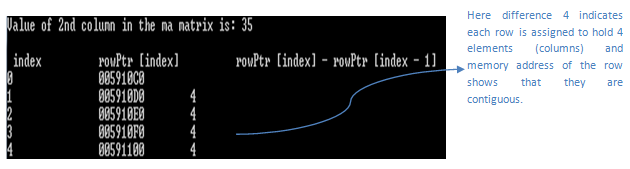In this method, we can see that we have only two calls for malloc – one for allocating memory for whole array and one for allocating memory to the row pointer. Hence this method is more efficient than 3rd method in terms of memory allocation at contiguous locations and call to malloc.

Above methods of allocating memory can be used for any dimensions of array and using pointers to them.

Below diagram shows how above method of memory allocation works in the case of 3X2 matrix array.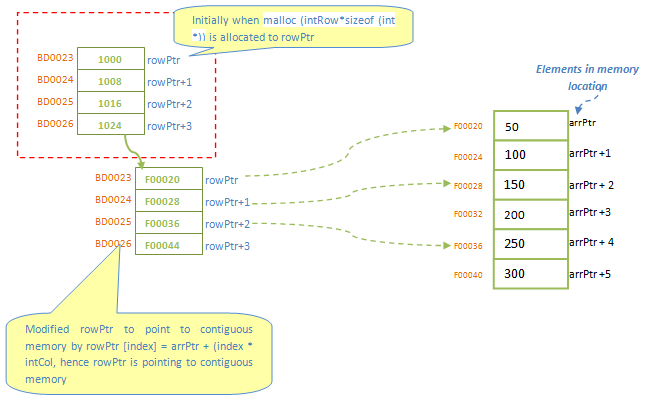Scroll to Top